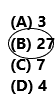# Texas Go Math Grade 3 Lesson 12.2 Answer Key Divide by 10

Refer to our Texas Go Math Grade 3 Answer Key Pdf to score good marks in the exams. Test yourself by practicing the problems from Texas Go Math Grade 3 Lesson 12.2 Answer Key Divide by 10.

## Texas Go Math Grade 3 Lesson 12.2 Answer Key Divide by 10

Essential Question
What strategies can you use to divide by 10?

There are 50 students going on a field trip to the Philadelphia Zoo. The students are separated into equal groups of 10 students each. How many groups of students are there?

• What do you need to find?
Answer: We need to find the number of groups of students is present.

• Circle the numbers you need to use.Explanation:
The total number of students going on a field trip=50
The number of students is divided into groups each=10
Therefore, divide 50 by 10=50/10=5 groups.

One Way
Use repeated subtraction.

• Subtract 10 until you reach 0.
• Count the number of times you subtract 10.You subtracted lo fIve times. 50 ÷ 10 = _5_
So, there are _5__ groups of 10 students.

Math talk
Mathematical Processes
How is counting on a number line to divide by 10 different from counting on a number line to multiply by 10?

Other Ways

A. Use a number line.

• Start at 50 and count back by 10s until you reach 0.
• Count the number of times you jumped back 10.

You jumped back by 10 five times.
50 ÷ 10 = _5__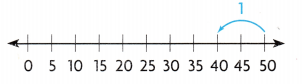B. Use a multiplication table.
Divide. 50 ÷ 10 = __5_
Since division is the opposite of multiplication, you can use a multiplication table to find a quotient.
Think of a related multiplication fact.
_5_ × 10 = 50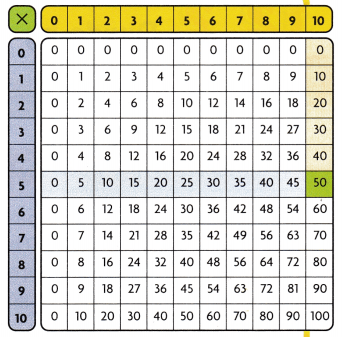Step 1
Find the factor, 10, in the top row.

Step 2
Look down to find the product, 50.

Step 3
Look left to find the unknown factor, _5__
Since _5__ × 10 = 50, then 50 ÷ 10 = _5__.

Math talk
Mathematical Processes
Describe two other ways to find 30 ÷ 10.

Share and Show

Question 1.
Use repeated subtraction to find 30 ÷ 1o. ____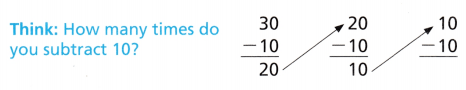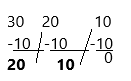{we subtracted 10, three times to get 0}
Explanation:
30/10=3.
A. multiplication.
Since division is the opposite of multiplication, you can use a multiplication table to find a quotient.
Think of a related multiplication fact.
3*10=30.
B. Use a number line.

• Start at 30 and count back by 10s until you reach 0.
• Count the number of times you jumped back 10.

You jumped back by 10 three times.
30/10=3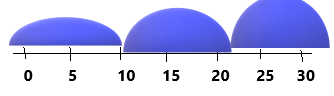Find the unknown factor and quotient.

Question 2.
10 × ___ = 40 ___ = 40 ÷ 10
10 × 4 = 40   4 = 40 ÷ 10
Explanation:
Since division is the opposite of multiplication, you can use a multiplication table to find a quotient.
Think of a related multiplication fact.
If we multiply with 4 with 10 then we get 40 and if we divide 40 by 10 then we get 4.

Question 3.
10 × ___ = 90 90 ÷ 10 = ____
10 × 9 = 90 90 ÷ 10 = 9
Explanation:
Since division is the opposite of multiplication, you can use a multiplication table to find a quotient.
Think of a related multiplication fact.
If we multiply with 10 with 9 then we get 90 and if we divide 90 by 10 then we get 9.

Problem Solving

H.O.T. Algebra Write>, <,or =.

Question 4.
10 ÷ 14 × 10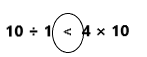Explanation:
Here, if we solve the above expression then we get the answers10 and 40.
In the question asked was write the symbol which is greater, lesser, or equal to.
According to the expression 10 is lesser than 40. so less than the (<) symbol is used.

Question 5.
17 – 618 ÷ 2Explanation:
Here, if we solve the above expression then we get the answers 11 and 9.
In the question asked was write the symbol which is greater, lesser, or equal to.
According to the expression 11 is greater than 9. so greater than the (>) symbol is used.

Question 6.
4 × 48 + 8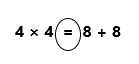Explanation:
Here, if we solve the above expression then we get the answers 16 and 16.
In the question asked was write the symbol which is greater, lesser, or equal to.
According to the expression 16 is equal to 16. so equal to (=) symbol is used.

Question 7.
Marie has loo packages of paper to pack evenly into 10 boxes. How many packages of paper did Marie put into each box?
Explanation:
Total number of packages of paper of Marie have=100
Number of boxes are there to pack=10
We need to find out the number of packages of paper did Marie put into each box.
Therefore, dividing 100 by 10 that means 100÷10=10 packages of paper had put in each box.

Problem Solving

Use the pictograph for 8-10.

Question 8.
Lyle wants to add penguins to the pictograph. There are 30 stickers of penguins. How many symbols should Lyle draw for penguins?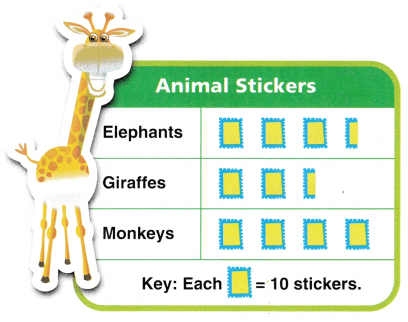Explanation:
Given question: Lyle wants to add a penguin in the given pictograph.
Number of stickers Lyle have=30
The number of stickers symbol is having=10
The number of stickers should Lyle draw for penguins=30÷10=3.
3 stickers should draw for penguins.

Question 9.
Multi-Step Pose a Problem Write a word problem using information from the pictograph. Then solve your problem.

Question 10.
Multi-Step Lena wants to put the monkey stickers in an album. She says she will use more pages if she puts 5 stickers on a page instead of 10 stickers on a page. Is she correct? Explain.Answer: Yes, she is correct. If she uses fewer stickers on the page then she takes more pages to stick that stickers. And if she uses more stickers on a page she takes fewer pages.

Question 11.
H.O.T. Explain how a division problem is like an unknown-factor problem.
First of all, we need to know what is a factor.
Factor: A whole number that divides exactly into another number.
For example, 3×5=15Now we can write factors 3×5=15
5×3=15
15÷5=3
15÷3=5.
You sometimes have to divide to find the unknown factor.

Fill in the bubble for the correct answer choice. You may use a division strategy to help you solve.

Question 12.
Ava smells dog shampoos. During 10 days, she smelled 40 different shampoos. She smelled the same number of shampoos each day. How many different shampoos did Ava smell each day?
(A) 4
(B) 10
(C) 14
(D) 40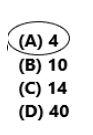Explanation:
Number of days Ava smells dog shampoos=10
Number of different shampoos she smelled=40
She smells the same number of shampoos each day, and moreover, we need to find out the number of different shampoos smells each day=40÷10=4
Therefore 4 different shampoos she smells each day.
Question 13.
Analyze Samantha jumps rope for 10 minutes every day. One day, she jumped 90 times in 10 minutes. She did the same number of jumps in each minute. How many jumps did Samantha do each minute?
(A) 10
(B) 90
(C) 9
(D) 900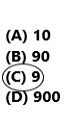Explanation:
Total number of minutes did Samantha jumps rope every day=10
Number of times she jumped rope in 10 minutes=90
She repeats the same number of jumps every minute, for that we need to find out the number of jumps did Samantha did every minute=90÷10=9
Therefore, 9 jumps she did every minute.

Question 14.
Multi-Step Elijah wrote his name in 15 school shirts. Cora wrote her name in 15 school shirts. Together they labeled 10 shirts each day. On how many days did Elijah and Cora label shirts?
(A) 10
(B) 3
(C) 15
(D) 30Explanation:
Total number of school shirts=30
Number of shirts elijah wrote his name=15
Number of shirts cora wrote her name=15
Number of shirts together they labelled each day=10
We need to find out the number of days they had taken to label the shirts.
Therefore, elijah shirts+cora shirts÷ shirts together they labelled each day.
15+15÷10=30÷10=3.
Texas Test Prep

Question 15.
Miles has 100¢ in dimes. How many dimes does Miles have?
(A) 1
(B) 100
(C) 10
(D) 4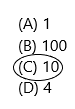Explanation:
Each dime is worth $0.10—that is the value of one dime. To find the total value of the mile of 100 dimes, multiply 100 by$0.10 to get $10. The number of dimes times the value of each dime equals the total value of the dimes. number·value=totalvalue. Therefore, 100$×\$0.10=10.

### Texas Go Math Grade 3 Lesson 12.2 Homework and Practice Answer Key

Use repeated subtraction or a number line to divide.

Question 1.
60 ÷ 10 = ___
Explanation: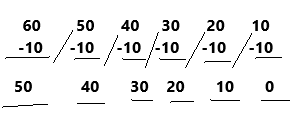If we divide 60 by 10 then we will get 6.
Cancel zeros then we have 6/1=6.

Question 2.
40 ÷ 10 = ___
Explanation: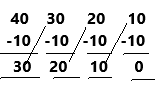If we divide 40 by 10 then we will get 4.
Cancel zeros then we have 4/1=4.

Problem Solving

Use the pictograph for 3-5.

Question 3.
Write a division sentence to show how many packs of sports cards Luke has.
Explanation:
The question asked was the number of packs of sports cards luke has. For this, we need to write a division equation.
By using pictographs: Luke is having 5 packs and each pack has 10 cards
From this we can write luke is having 5×10=50 cards
Therefore, the division sentence could be 50÷10.Question 4.
Joanna has 40 sports cards. Write a division sentence to show how many Hakim packs of sports cards she has.
Explanation:
The question asked was the number of packs of sports cards Hakim has. For this, we need to write a division equation.
By using pictographs: Hakim is having 5 packs and each pack has 10 cards
From this we can write luke is having 5×10=50 cards
Therefore, the division sentence could be 50÷10.

Question 5.
Write a division sentence to show how many packs of sports cards Luke and Mavis have in all. Explain.
Explanation:
By using pictographs: Luke is having 5 packs and each pack is having 10 cards=5*10=50
Mavis is having 3 packs and each pack is having 10 cards=3*10=30
Together they are having 50+30=80.
Now from this, we can write the division equation=80÷ 10.

Texas Test Prep

Question 6.
Walter has 80 pennies. He arranges the pennies in stacks of 10. How many stacks of pennies does Walter have?
(A) 10
(B) 90
(C) 70
(D) 8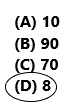Explanation:
Total number of pennies Walter is having=80
Number of pennies he arranged like a stack=10
Now we need to find the number of stacks of pennies does Walter have= 80÷10=8
Therefore, 8 stacks are there.

Question 7.
There are 40 students going on a class trip. Each van seats 10 students. How many vans are needed for the class trip?
(A) 40
(B) 4
(C) 3
(D) 5Explanation:
Number of students going on a class trip=40
Number of seats in a van=10
Now we need to find the number of vans needed for the class trip=40÷10=4
Therefore, 4 vans are needed.

Question 8.
There are 70 pieces of chalk in a box. If each of 10 students gets an equal number of chalk pieces, how many pieces of chalk does each student get?
(A) 80
(B) 10
(C) 7
(D) 8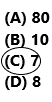Explanation:
Total number of chalk pieces in a box=70

The number of students gets an equal number of chalk pieces each=10
Now the number of pieces of chalk does each student get=70÷10=7
Therefore, 7 pieces of chalk will get to every student.

Question 10.
Multi-Step Peyton has 32 cubes. Myra has 18 cubes. If both students use all of the cubes to make trains with 10 cubes, how many trains can they make?
(A) 3
(B) 5
(C) 50
(D) 4Explanation:
The number of cubes Peyton is having=32.
The number of cubes Myra is having=18.
Together they are having=32+18=50 cubes.
Both students are using cubes to make train=10
We need to find out the number of trains they can make=50÷10=5
Therefore, they can make 5 trains.

Question 11.
Multi-Step Roshan has 37 dimes. If she makes stacks of 10 coins, how many coins will Roshan have leftover?
(A) 3
(B) 27
(C) 7
(D) 4